# Acta Universitatis Danubius. Œconomica, Vol 11, No 4 (2015)

Mathematical and Quantative Methods

A Study of Integers Using Software Tools – III

Cătălin Angelo Ioan1, Alin Cristian Ioan2

Abstract: The paper deals with a generalization of polite numbers that is of those numbers that are sums of consecutive integers to the numbers called almost polite numbers of order p,m which can be written in m ways as sum of two or more consecutive of same powers p of natural numbers.

Keywords: polite numbers; divisibility

1 Introduction

Let note for any nN*, pN*, Sn,p=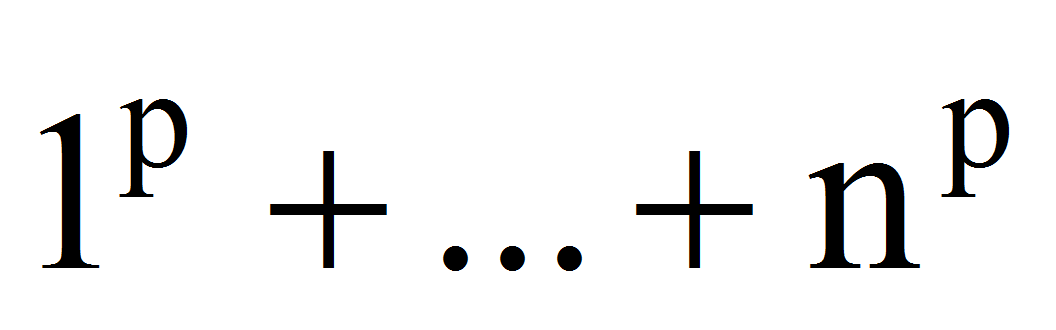and Sk,n,p=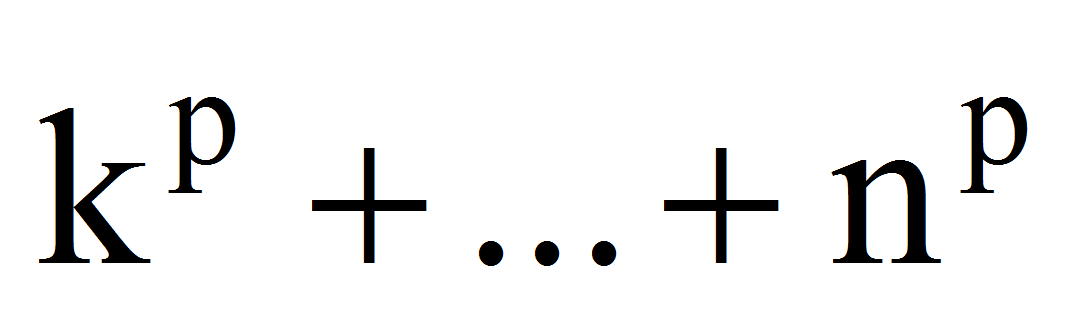=Sn,p-Sk-1,p, k=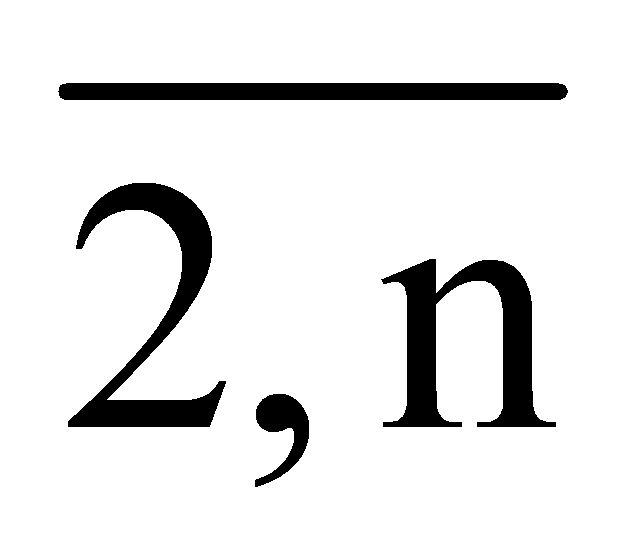.

It is well known that: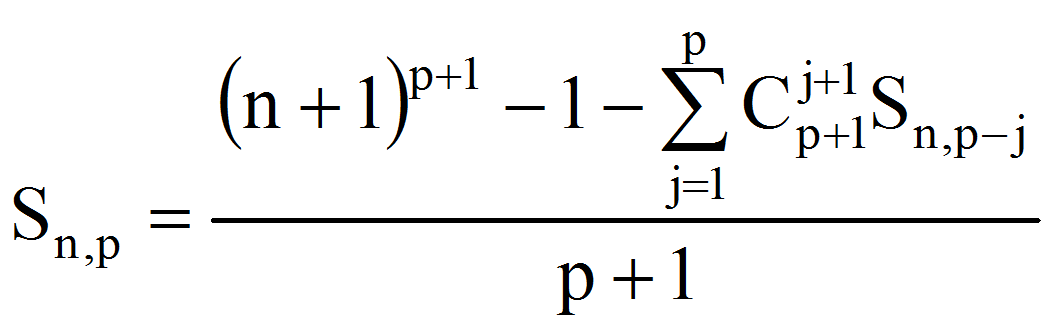and also: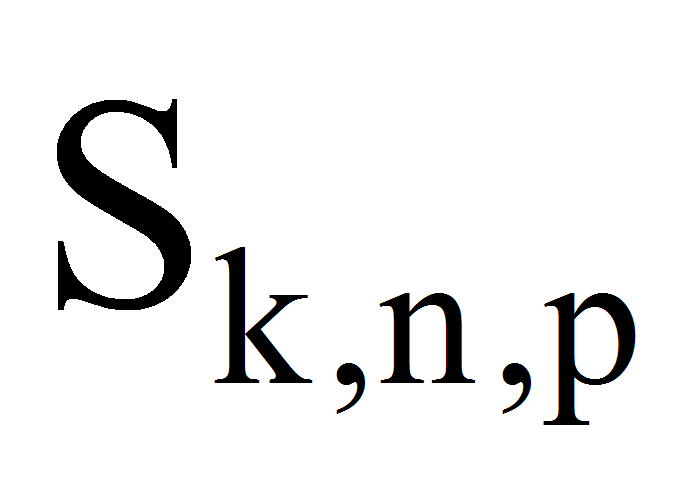=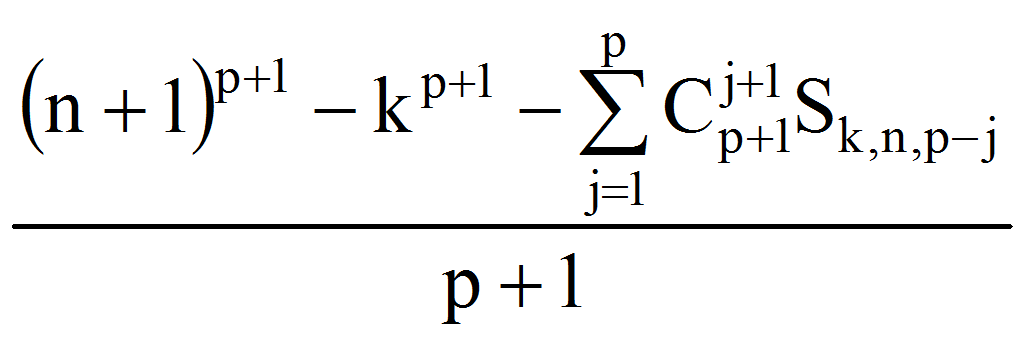It is easly to see that the first 10 sums are:

Sn,1=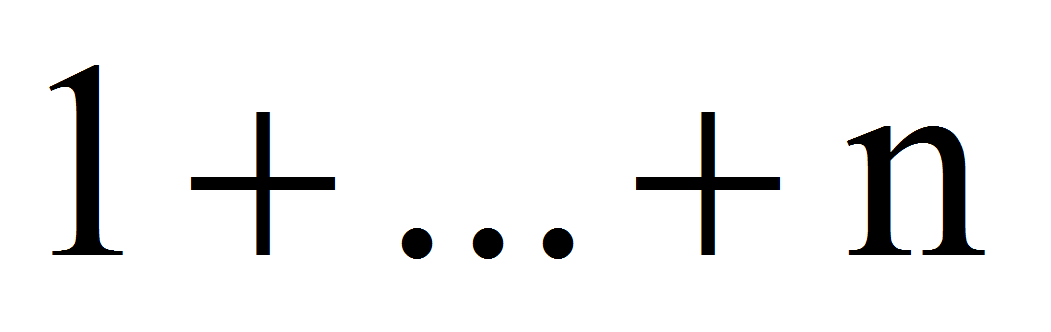=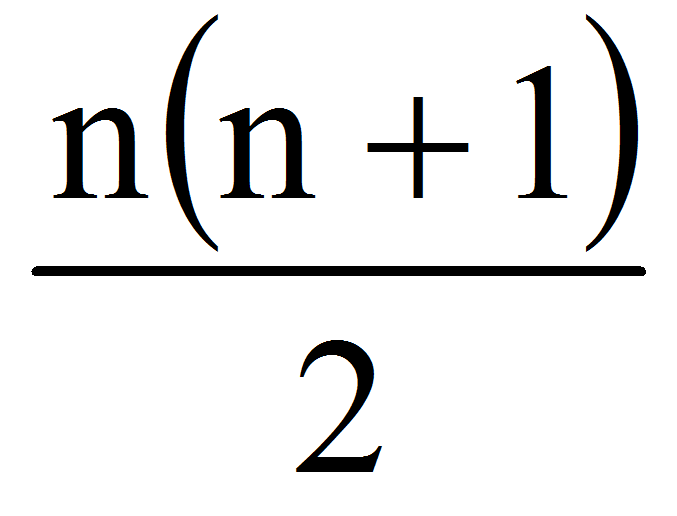Sn,2=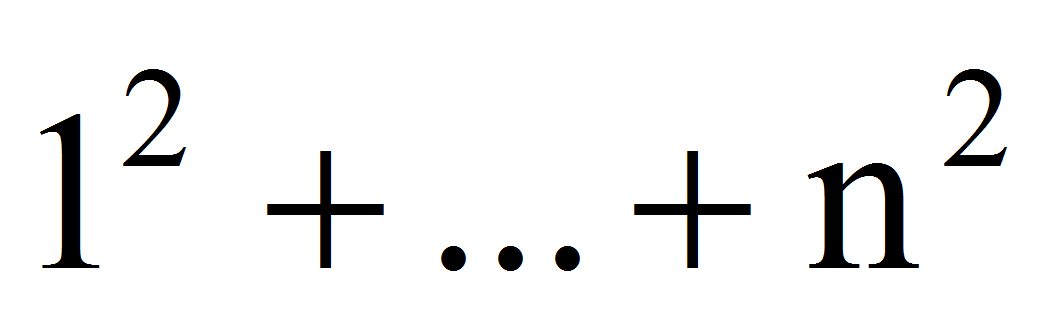=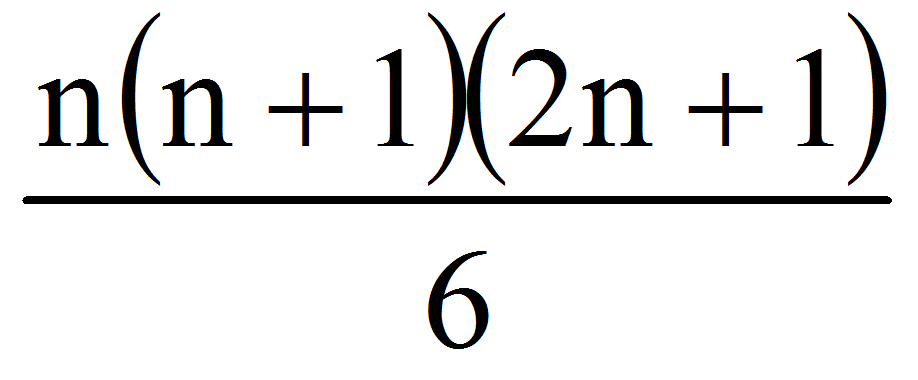Sn,3=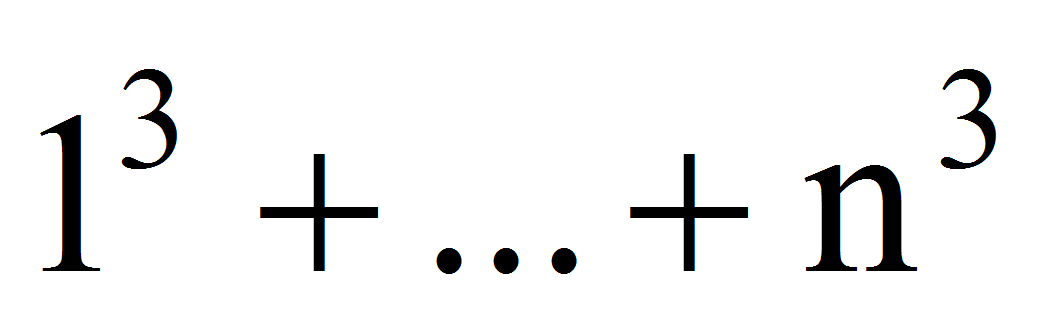=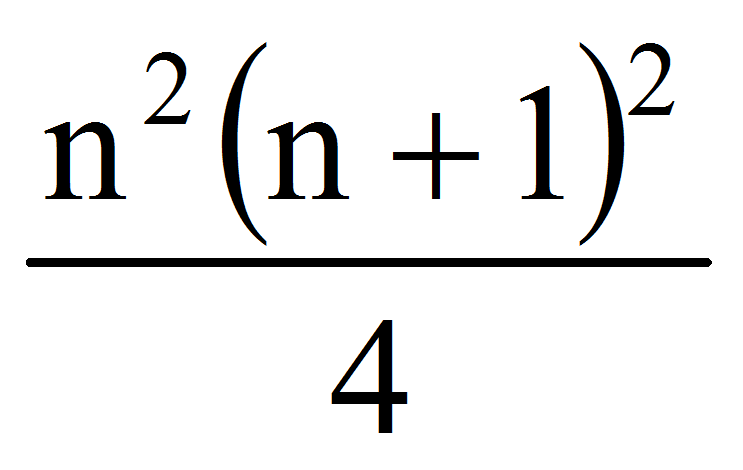Sn,4=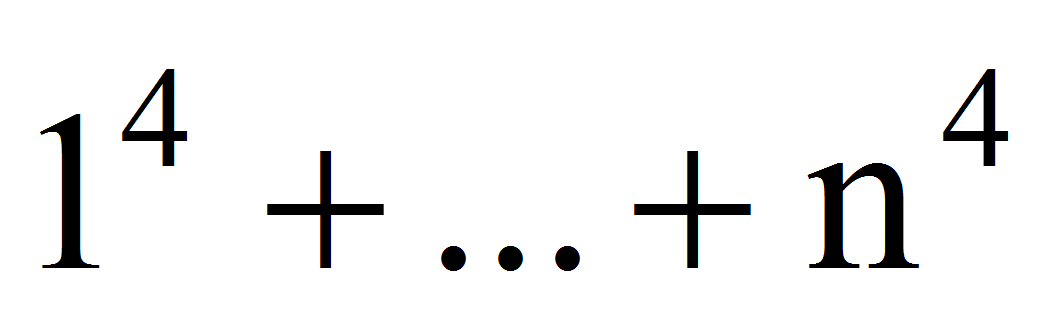=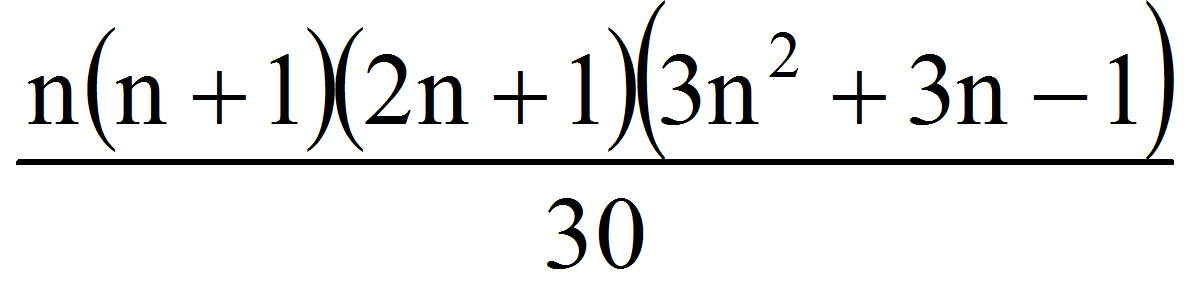Sn,5=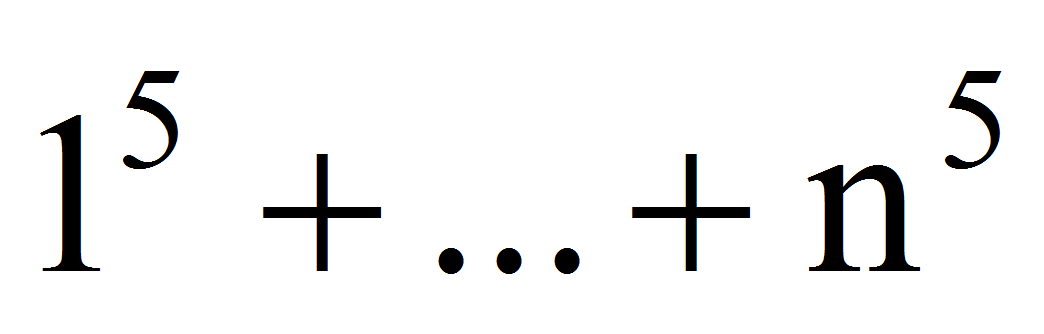=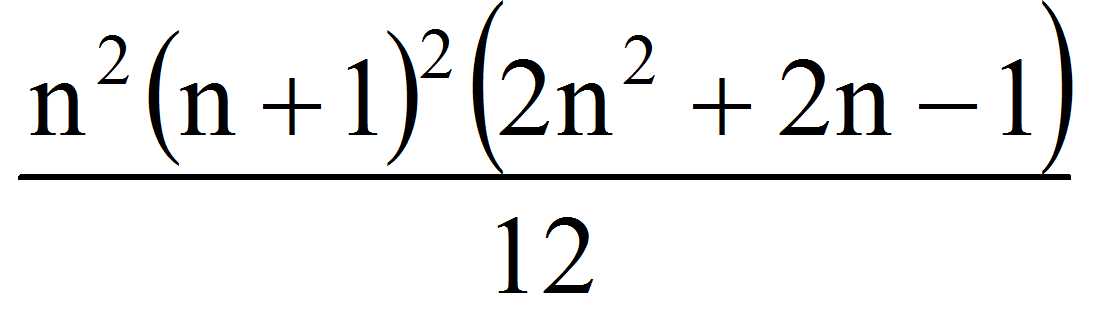Sn,6=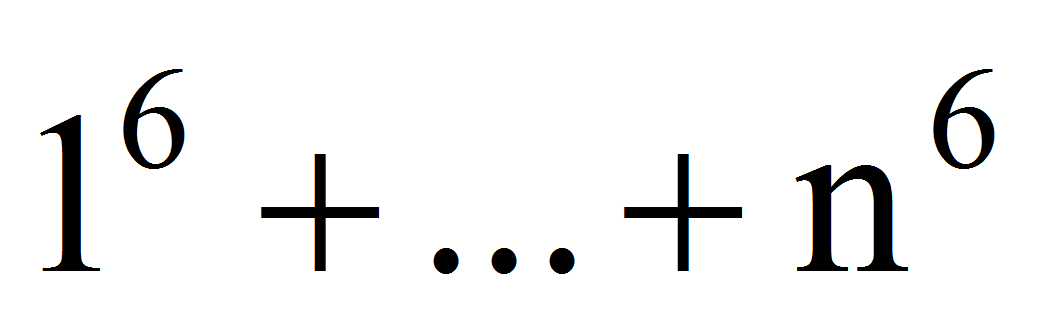=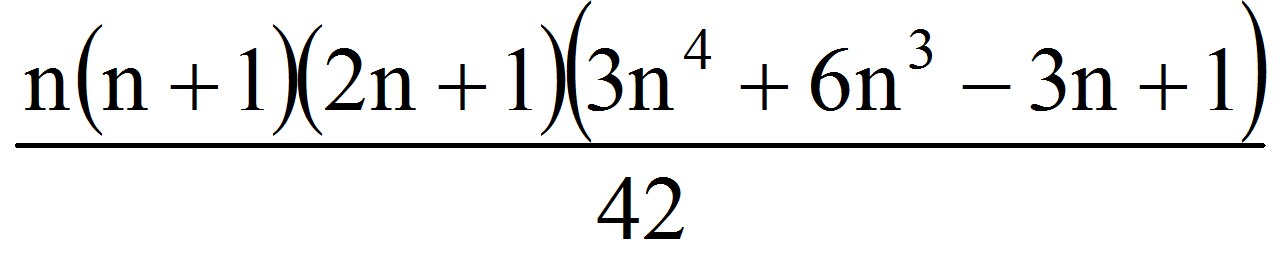Sn,7=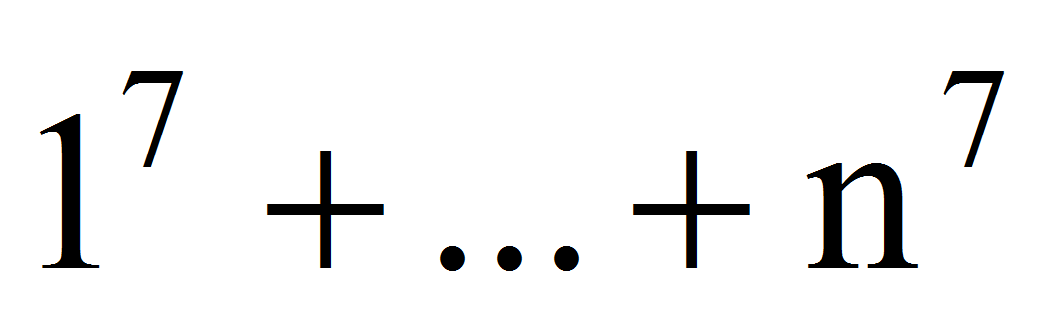=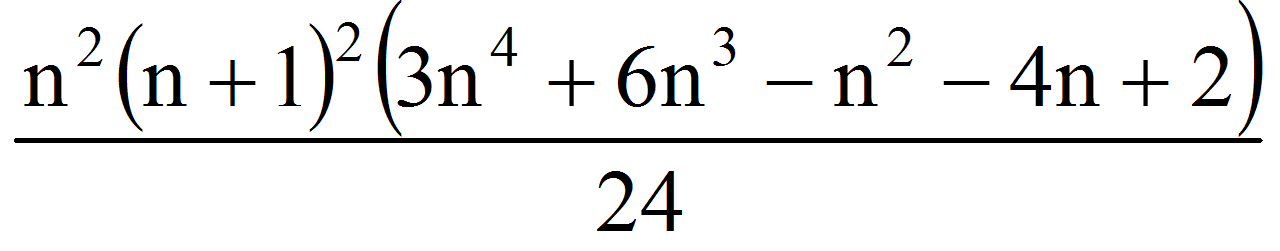Sn,8=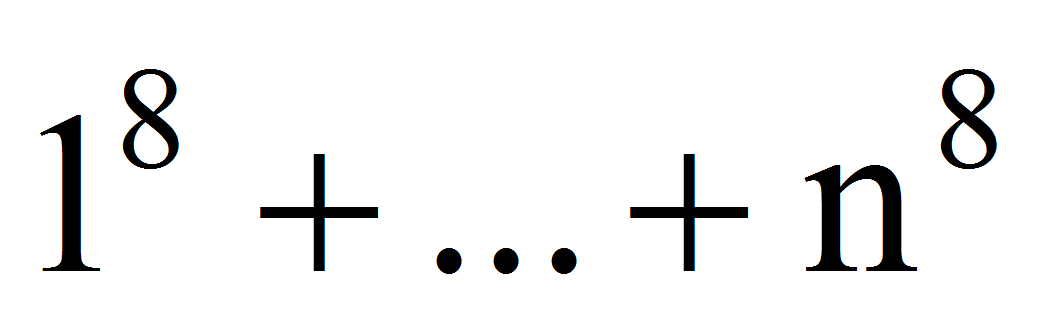=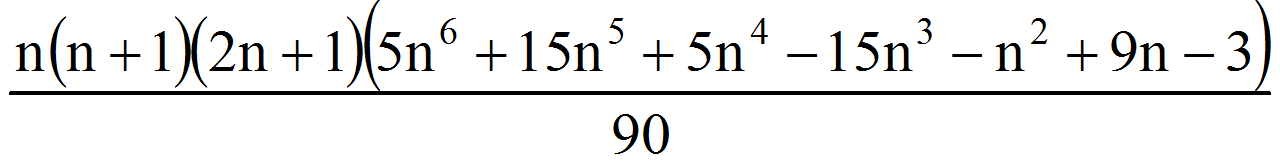Sn,9=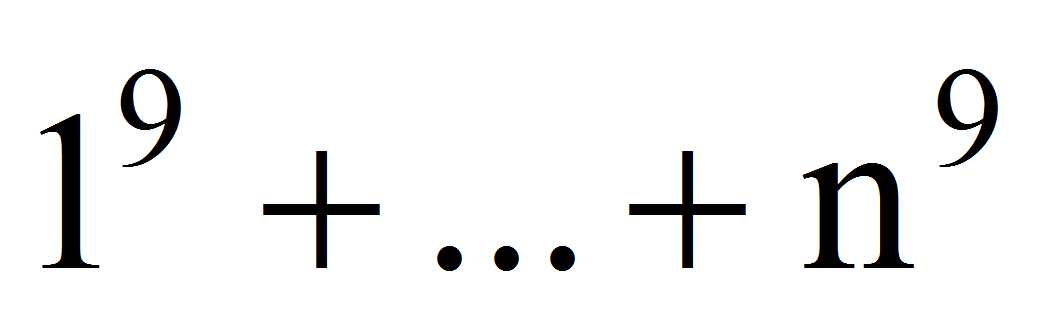=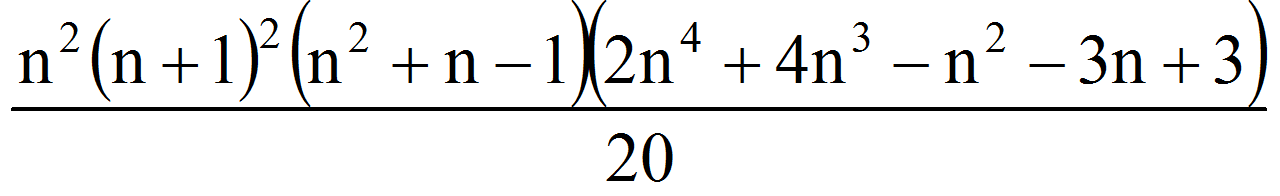Sn,10=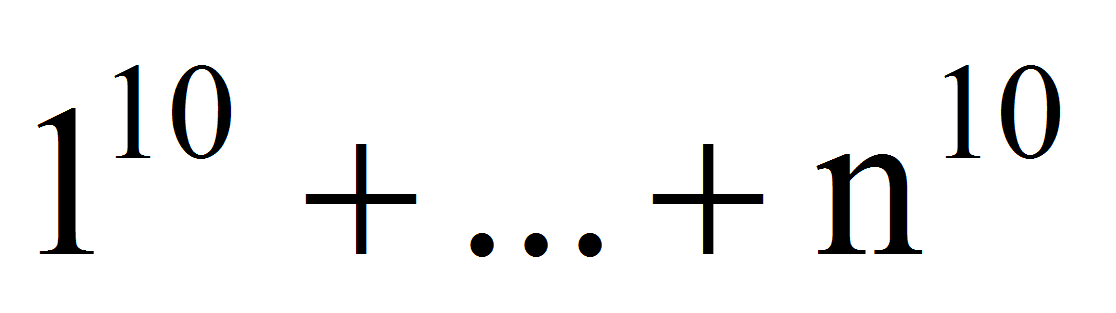=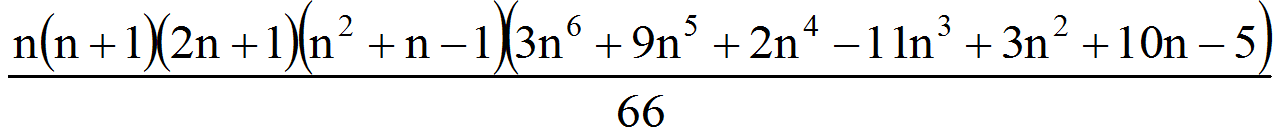All over in this paper, the software presented was written in Wolfram Mathematica 9.0.

2 Almost Polite Numbers of Order p,m

A natural number N greather than 2 will be called almost polite number of order p if it can be written as sum of two or more consecutive of a same power p of natural numbers.

If N is odd it is natural that for N=2k+1 we have N=k+(k+1) therefore each odd natural number is polite of order 1.

A natural number N greather than 2 will be called almost polite number of order p,m if it can be written in m ways as a sum of two or more consecutive of same powers p of natural numbers.

Therefore N is a polite number of order p,m if:

N=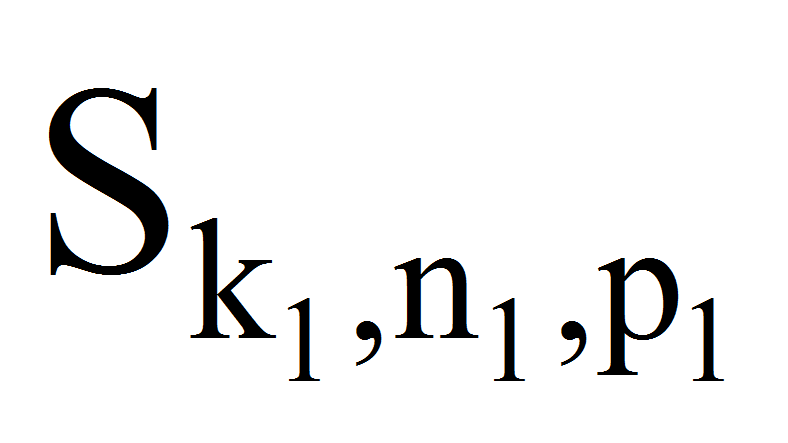=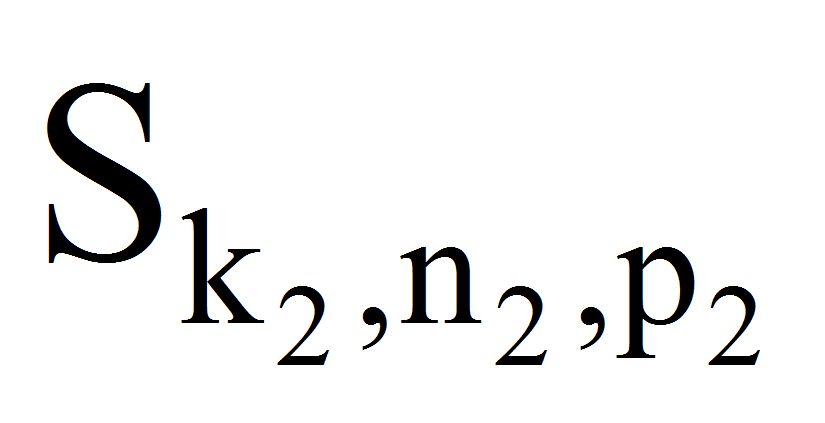=...=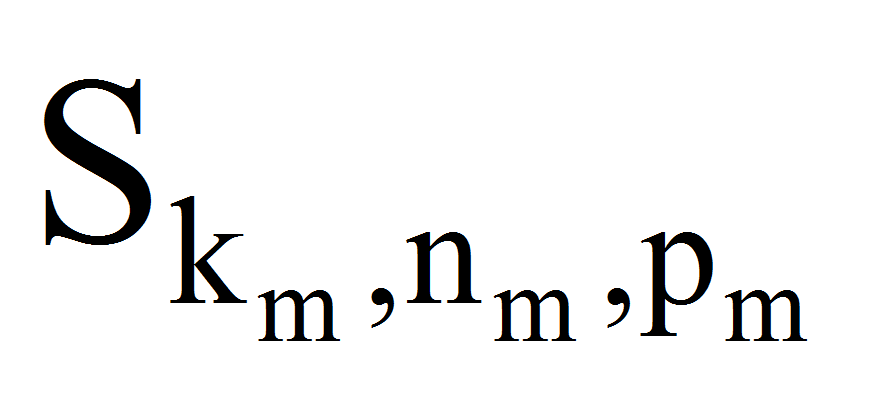with p1p2...pm

The software for determining the almost polite numbers of order p,m (m3) limited to 30000 and powers less than or equal with 10 is:

Clear["Global`*"];

limit=30000;

pmax=10;

nrorimax=3;

nrk=Table[i,{i,nrorimax}];

nrn=Table[i,{i,nrorimax}];

nrp=Table[i,{i,nrorimax}];

S=n;

(*The calculus of sums of powers from 1 to n*)

For[p=1,ppmax,p++,

suma=0;

For[j=1,jp,j++,suma=suma+Binomial[p+1,j+1]*S[p-j]];

S[p]=Factor[((n+1)^(p+1)-1-suma)/(p+1)]]

(*The calculus of sums of powers from k to n*)

For[p=1,ppmax,p++,sumpower[n_,p]=S[p]];

For[p=1,ppmax,p++,sumpowerkn[n_,k_,p]=Factor[Simplify[sumpower[n,p]-

sumpower[k-1,p]]]]

(*The analysis*)

For[number=2,numberlimit,number=number+1,nrori=0;

For[p=1,ppmax,p++,

For[n=2,nnumber^(1/p),n++,

For[k=1,kn-1,k++,

If[sumpowerkn[n,k,p]==number,nrori=nrori+1;nrk[[nrori]]=k;nrn[[nrori]]=n;

nrp[[nrori]]=p];

If[nrori2&& nrp[[nrori]]==nrp[[nrori-1]],nrori=nrori-1]]]];

If[nrorinrorimax,For[k=1,knrori,k++,Print[number,"=\[Sum](power=",nrp[[k]],") from ",nrk[[k]]," to ",nrn[[k]]]];Print[""]]]

We found (till 30000):

• 91=1+2+...+13=12+...+62=33+43

• 559=9+10+...+34=72+...+122=63+73

• 855=4+5+...+41=112+...+152=73+83

• 6985=9+10+...+118=202+...+302=93+...+133

• 19721=200+201+...+281=142+...+392=46+56

• 24979=12489+12490=622+...+672=54+...+104

• 29240=29+30+...+243=352+...+502=23+...+183

3. References

Adler, A. & Coury, J.E. (1995). The Theory of Numbers. London, UK: Jones and Bartlett Publishers International.

Baker, A. (1984). A Concise Introduction to the Theory of Numbers. Cambridge: Cambridge University Press.

Coman, M. (2013). Mathematical Encyclopedia of Integer Classes. Educational Publishers.

Guy, R.K. (1994). Unsolved Problems in Number Theory. Second Edition. New York: Springer Verlag.

Hardy, G.H. & Wright, E.M. (1975). Introduction to the Theory of Numbers. Fourth Edition. Oxford: Oxford University Press.

Krantz, S.G. (2001). Dictionary of Algebra, Arithmetic and Trigonometry. CRC Press.

Niven, I., Zuckerman, H.S. & Montgomery, H.L. (1991). An Introduction to the Theory of Numbers. Fifth Edition. New York: John Wiley & Sons, Inc.

Sierpinski, W. (1995). Elementary theory of numbers. Second Edition. Elsevier.

Wai, Y.P. (2008). Sums of Consecutive Integers. arXiv:math/0701149v1 [math.HO].

1 Associate Professor, PhD, Danubius University of Galati, Faculty of Economic Sciences, Romania, Address: 3 Galati Blvd, Galati, Romania, Tel.: +40372 361 102, Fax: +40372 361 290, Corresponding author: catalin_angelo_ioan@univ-danubius.ro.

2 Nicolae Oncescu College, Braila, Address: 1-3 ŞOS. Brăilei, City: Ianca, Brăila County, Tel.: +40239-668 494, E-mail: alincristianioan@yahoo.com.

AUDŒ, Vol. 11, no. 4, pp. 41-44

### Refbacks

• There are currently no refbacks.